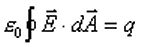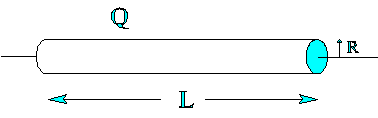Teaching Physics with the Physics Suite Edward F. Redish

Using Gauss's Law

Gauss's law is usually written as an equation in the form.

1. For this equation, specify what each term in this equation means and how it is to be calculated when doing some specific (but arbitrary - not a special case!) calculation.

A long thin cylindrical shell of length L and radius R with L>>R is uniformly covered with a charge Q. If we look for the field near to the cylinder somewhere about the middle, we can treat the cylinder as if it were an infinitely long cylinder. Using this assumption, we can calculate the magnitude and direction of the field at a point a distance d from the axis of the cylinder (outside the cylindrical shell, i.e., L>>d > R but d not very close to R) using Gauss's Law. Do so by explicitly following the steps below.1. Select an appropriate Gaussian surface. Explain why you chose it.
2. Carry out the integral on the left side of the equation, expressing it in terms of the unknown value of the magnitude of the E field.
3. What is the relevant value of q for your surface?
4. Use your results in (c) and (d) in the equation and solve for the magnitude of E

Note to the instructor: Gauss's Law can be very difficult for students in an introductory physics class. They are not comfortable with integrals. Even if they have studied integrals, calculating an integral symbolically and finding an unknown from under the integral is rarely done in a math class. This helps work through some of the barriers.

Not finding what you wanted? Check the Site Map for more information.